Next: Exercises Up: Central Potentials Previous: Hydrogen Atom

# Rydberg Formula

An electron in a given stationary state of a hydrogen atom, characterized by the quantum numbers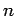,, and, should, in principle, remain in that state indefinitely. In practice, if the state is slightly perturbed--e.g., by interacting with a photon--then the electron can make a transition to another stationary state with different quantum numbers.

Suppose that an electron in a hydrogen atom makes a transition from an initial state whose radial quantum number is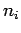to a final state whose radial quantum number is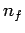. According to Eq. (676), the energy of the electron will change by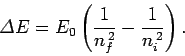(699)

If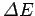is negative then we would expect the electron to emit a photon of frequency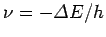[see Eq. (58)]. Likewise, ifis positive then the electron must absorb a photon of energy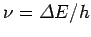. Given that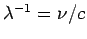, the possible wavelengths of the photons emitted by a hydrogen atom as its electron makes transitions between different energy levels are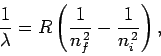(700)

where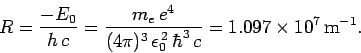(701)

Here, it is assumed that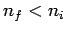. Note that the emission spectrum of hydrogen is quantized: i.e., a hydrogen atom can only emit photons with certain fixed set of wavelengths. Likewise, a hydrogen atom can only absorb photons which have the same fixed set of wavelengths. This set of wavelengths constitutes the characteristic emission/absorption spectrum of the hydrogen atom, and can be observed as spectral lines'' using a spectroscope.

Equation (700) is known as the Rydberg formula. Likewise,is called the Rydberg constant. The Rydberg formula was actually discovered empirically in the nineteenth century by spectroscopists, and was first explained theoretically by Bohr in 1913 using a primitive version of quantum mechanics. Transitions to the ground-state (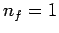) give rise to spectral lines in the ultraviolet band--this set of lines is called the Lyman series. Transitions to the first excited state (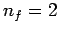) give rise to spectral lines in the visible band--this set of lines is called the Balmer series. Transitions to the second excited state (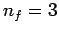) give rise to spectral lines in the infrared band--this set of lines is called the Paschen series, and so on.

SubsectionsNext: Exercises Up: Central Potentials Previous: Hydrogen Atom
Richard Fitzpatrick 2010-07-20